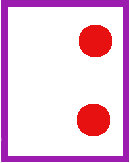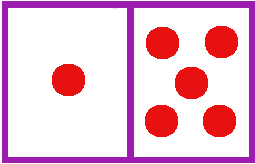# Order of

## Lesson Title:

Order of Operations Introduction

## Abstract

This lesson is about evaluating numerical expressions, and it was designed for adult learners who are preparing to take their High School Equivalency tests. This course will help the students evaluate numerical expressions correctly by following the correct order of operations, focusing on multiplication and addition and subtraction for this introductory lesson.

.

## Learner Audience / Primary Users

This lesson was designed for adult learners that are preparing to take their High School Equivalency tests, and instructors as well, or anyone who wishes to understand the order of operations.

## Educational Use

• Curriculum / Instruction

## College & Career Readiness Standards (CCRS) Alignment

• Subject: Mathematics
• Domain: Operations and Algebraic Thinking
• Standard: Use parentheses, brackets, or braces in numerical expressions, and evaluate expressions with these symbols. (5.OA.1)
• Domain: Equations and expressions
• Standard Description:  Write and evaluate numerical expressions involving whole-number exponents. (6.EE.1)

English

## Material Type

• Instructional Material
• Homework and Assignments
• Images and Videos
• Diagnostic, formative and final assessments

## Learning Goals

The purpose of this lesson is for learners to:

• Identify the need for having an order of operations in evaluating a numerical expression (figuring out the answer to a problem).
• Identify the need for having an order of operations in evaluating a numerical expression (figuring out the answer to a problem).
• Identify which operations have priority when evaluating a numerical expression.
• Apply the order of operations correctly when evaluating numerical expressions.
• Simplify their answers as much as possible when evaluating numerical expressions.

## Keywords

• Designers for Learning
• Exponents
• Grouping symbols
• Numerical expressions
• Order of operations
• PEMDAS

35 minutes

## Prior Knowledge

Students should be able to:

• Work with the basic arithmetic operations.
• Use parentheses, brackets, or braces in numerical expressions and evaluate expressions with these symbols.

## Required Resources

Depending on the resources available, this course can be delivered as a fully online course, a face-to-face course, or a hybrid course.

• Access to an electronic device which includes: computers, smartphones and tablets
• Whiteboard and whiteboard markers
• Paper
• Pencil
• Eraser

• Andres Chan -- remix by Susan Jones

## Learning Objectives

By the end of this lesson, the learner should be able to:

• Identify which operations have priority when evaluating a numerical expression.
• Apply the order of operations correctly when evaluating numerical expressions.
• Simplify their answers as much as possible when evaluating numerical expressions.

## Lesson Topics

Key topics covered in this lesson include:

• Evaluating numerical expressions
• Order of operations
• Grouping symbols and exponents

## Context Summary

This course will engage students to one of the fundamental skills in Arithmetic, which is the order of operations. By learning the correct order in which a numerical expression should be solved, the students will be able to apply this knowledge in future courses in Algebra, Geometry and Precalculus.

## Relevance to Practice

Many people are not conscious that there are conventions applied to solve numerical problems involving more than one operation. This lesson aims to provide the students with the knowledge to apply the most basic convention -- doing multiplication & subtraction before addition or subtraction --    and connecting the conventions to practical applications.

## Key Terms and Concepts

• Numerical expression
• Evaluating numerical expressions
• Grouping symbols
• Order of operations
• PEMDAS (parentheses, exponents, multiplication, division, addition, subtraction)
• Simplifying expressions

## Instructional Strategies and Activities

### Warm-Up

Time: 1 minutes

TTime: 2 minutes

Match the pictures to the math problems here, or have students create representations with manipulatives.

You can create your own with icons or pictures that will have meaning to your students (or humor), or with manipulatives they are familiar with.

Have students match different representations of this multiplication and addition problem using images or manipulatives.   Students can create their own representations, as well.

2 x 6        2 + 6Ask:   What happens when we have to mix them up?? What happens when we have to add and multiply?

:I can't figure out how to delete this video.   It is much more comprehensive than this lesson and compresses the whole order of operations into one video.

• The teacher should make sure that the students understand that multiplication and division have the same priority, and when

### Introduction

Time:  minute

When we are doing different operations, we need to be able to:

(Goals of this lesson):

• Identify which operations have priority when evaluating a numerical expression.

• Apply the order of operations correctly when evaluating numerical expressions.

• Simplify their answers as much as possible when evaluating numerical expressions.

### Presentation / Modeling / Demonstration

Time: 12 minutes

I remixed the introductory slideshow here:  https://drive.google.com/open?id=0B11LhZpTaZQJZU1xaTk2bnN3RkE  ….

• Students watch video at https://www.youtube.com/watch?v=rluT-dtifO8   (4 minutes) or use this as a guide, so that students can engage and discuss these ideas.

• Students watch this movie to see a useful way to do more complex problems without losing track of details: https://www.youtube.com/watch?v=zBNiB-G1vlI      (2 minutes)

• Explain that multiplication and division have the same priority, and when they face a problem with both multiplication and division or division and multiplication one next to the other, they should solve the problem from left to right. For example:   12 / 4 x 3 =  12 / 4 x 3 = 3 x 3 = 9

• The same rule applies when there is an addition and subtraction, one next to the other. You should solve the problem from left to right. For example: 16 - 4 + 12 = 16 - 4 + 12 = 12 + 12 = 24

### Guided Practice

Time: 5 minutes

The students will play an interactive game to test their mastery of the order of operations. The game has different levels, and if the student has difficulty getting the right answers, there is an option for hints about PEMDAS.

When a student makes a mistake, the game gives an instant feedback on what was wrong.

Exploring Order of Operations - Use It! (link to the interactive game)

### Evaluation

Time: 10 minutes

You can generate practice sets at http://www.math-aids.com/Order_of_Operations/   including

Without division at http://www.math-aids.com/Order_of_Operations/Order_of_Operations_No_Division.html  .   or create your own.

Typical problems:

9 + 5 + 3 x 10   (= 44)

3 + 8 x 5 - 3   (40)

6 x 5 + 3 x 3  (39).

Part 3: Supplementary Resources & References

http://www.saddleback.edu/faculty/lperez/algebra2go/prealgebra/index.html   (includes order of operations under “Multiplication and Division” with English and Spanish versions. )